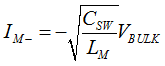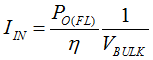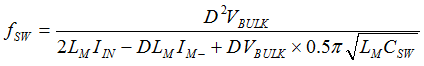JAJSE40A October   2017  – February 2018

PRODUCTION DATA.

1. 特長
2. アプリケーション
3. 概要
1.     Device Images
4. 改訂履歴
5. Pin Configuration and Functions
6. Specifications
7. Detailed Description
1. 7.1 Overview
2. 7.2 Functional Block Diagram
3. 7.3 Detailed Pin Description
4. 7.4 Device Functional Modes
8. Application and Implementation
1. 8.1 Application Information
2. 8.2 Typical Application Circuit
1. 8.2.1 Design Requirements
2. 8.2.2 Detailed Design Procedure
3. 8.2.3 Application Curves
9. Power Supply Recommendations
10. 10Layout
1. 10.1 Layout Guidelines
2. 10.2 Layout Example
11. 11デバイスおよびドキュメントのサポート
12. 12メカニカル、パッケージ、および注文情報

#### パッケージ・オプション

デバイスごとのパッケージ図は、PDF版データシートをご参照ください。

• D|16
• RTE|16
• RTE|16

#### 8.2.2.2.3 Primary Turns (NP)

The turn number on the primary side of the transformer (NP) is determined by two design considerations:

1. The maximum flux density (BMAX) must be kept below the saturation limit (BSAT) of the magnetic core under the highest peak magnetizing current (IM+(MAX)) condition, a given cross-section area (AE) of the core geometry, and highest core temperature. When IFB = 0 A, such as VO soft-start or step-up load transient, the peak magnetizing current reaches IM+(MAX), since VCST = VCST(MAX) in those conditions. IM+(MAX) can be calculated based on the output power triggering an OPP fault (PO(OPP)) with VCST = VCST(OPP1) at VBULK(MIN). After NP is chosen, NS can be calculated through NPS.
2. Equation 24.Equation 25.3. The AC flux density (ΔB) affects the core loss of a transformer. For a transition-mode active clamp flyback, the core loss at high line is usually highest, since the switching frequency is highest and duty cycle is smallest for a given load condition. The following equation is the ΔB calculation including the contribution of negative magnetizing current (IM-), used to put into the Steinmetz equation for more accurate core loss estimation. For VBULK ≥ NPS(VO+VF), IM- is calculated with VBULK divided by the characteristic impedance of LM and the lumped time-related switch-node capacitance (CSW). The expression of fSW is derived based on the triangular approximation of the magnetizing current, which also considers IM- effect over wide AC line condition.
4. Equation 26.Equation 27.Equation 28.Equation 29.Equation 30.Equation 31.